# 2.7 Solve linear inequalities  (Page 2/8)

 Page 2 / 8

The inequality $x\le 1$ means all numbers less than or equal to 1. There is no lower end to those numbers. We write $x\le 1$ in interval notation as $\left(\text{−}\infty ,1\right]$ . The symbol $\text{−}\infty$ is read as ‘negative infinity’. [link] shows both the number line and interval notation.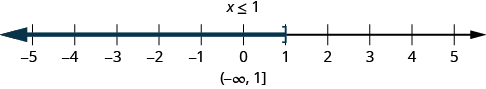The inequality x ≤ 1 is graphed on this number line and written in interval notation.

## Inequalities, number lines, and interval notation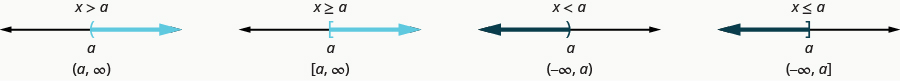Did you notice how the parenthesis or bracket in the interval notation matches the symbol at the endpoint of the arrow? These relationships are shown in [link] .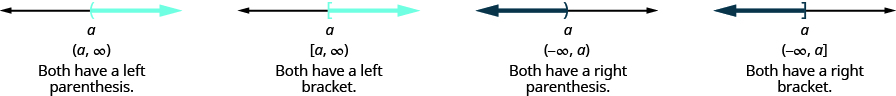The notation for inequalities on a number line and in interval notation use similar symbols to express the endpoints of intervals.

Graph on the number line and write in interval notation.

$x\ge -3$ $x<2.5$ $x\le -\frac{3}{5}$

## Solution

1.Shade to the right of $-3$ , and put a bracket at $-3$ .Write in interval notation.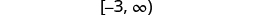2.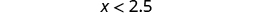Shade to the left of $2.5$ , and put a parenthesis at $2.5$ .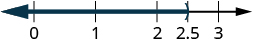Write in interval notation.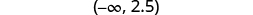3.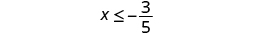Shade to the left of $-\frac{3}{5}$ , and put a bracket at $-\frac{3}{5}$ .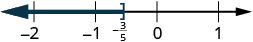Write in interval notation.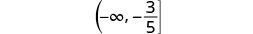Graph on the number line and write in interval notation:

$x>2$ $x\le -1.5$ $x\ge \frac{3}{4}$

1.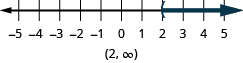2.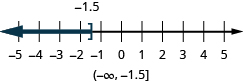3.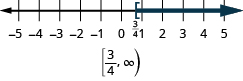Graph on the number line and write in interval notation:

$x\le -4$ $x\ge 0.5$ $x<-\frac{2}{3}$

1.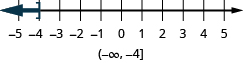2.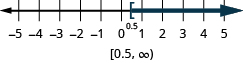3.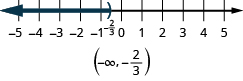## Solve inequalities using the subtraction and addition properties of inequality

The Subtraction and Addition Properties of Equality state that if two quantities are equal, when we add or subtract the same amount from both quantities, the results will be equal.

## Properties of equality

$\begin{array}{cccc}\mathbf{\text{Subtraction Property of Equality}}\hfill & & & \mathbf{\text{Addition Property of Equality}}\hfill \\ \text{For any numbers}\phantom{\rule{0.2em}{0ex}}a,b,\text{and}\phantom{\rule{0.2em}{0ex}}c,\hfill & & & \text{For any numbers}\phantom{\rule{0.2em}{0ex}}a,b,\text{and}\phantom{\rule{0.2em}{0ex}}c,\hfill \\ \begin{array}{cccc}\text{if}\hfill & \hfill a& =\hfill & b,\hfill \\ \text{then}\hfill & \hfill a-c& =\hfill & b-c.\hfill \end{array}\hfill & & & \begin{array}{cccc}\text{if}\hfill & \hfill a& =\hfill & b,\hfill \\ \text{then}\hfill & \hfill a+c& =\hfill & b+c.\hfill \end{array}\hfill \end{array}$

Similar properties hold true for inequalities.

 For example, we know that −4 is less than 2.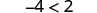If we subtract 5 from both quantities, is the left side still less than the right side?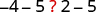We get −9 on the left and −3 on the right.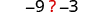And we know −9 is less than −3.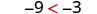The inequality sign stayed the same.

Similarly we could show that the inequality also stays the same for addition.

## Properties of inequality

$\begin{array}{cccc}\mathbf{\text{Subtraction Property of Inequality}}\hfill & & & \mathbf{\text{Addition Property of Inequality}}\hfill \\ \text{For any numbers}\phantom{\rule{0.2em}{0ex}}a,b,\text{and}\phantom{\rule{0.2em}{0ex}}c,\hfill & & & \text{For any numbers}\phantom{\rule{0.2em}{0ex}}a,b,\text{and}\phantom{\rule{0.2em}{0ex}}c,\hfill \\ \begin{array}{cccccc}& & \text{if}\hfill & \hfill a& <\hfill & b\hfill \\ & & \text{then}\hfill & \hfill a-c& <\hfill & b-c.\hfill \\ \\ & & \text{if}\hfill & \hfill a& >\hfill & b\hfill \\ & & \text{then}\hfill & \hfill a-c& >\hfill & b-c.\hfill \end{array}\hfill & & & \begin{array}{cccccc}& & \text{if}\hfill & \hfill a& <\hfill & b\hfill \\ & & \text{then}\hfill & \hfill a+c& <\hfill & b+c.\hfill \\ \\ & & \text{if}\hfill & \hfill a& >\hfill & b\hfill \\ & & \text{then}\hfill & \hfill a+c& >\hfill & b+c.\hfill \end{array}\hfill \end{array}$

We use these properties to solve inequalities, taking the same steps we used to solve equations. Solving the inequality $x+5>9$ , the steps would look like this:

$\begin{array}{cccccc}& & & \hfill x+5& >\hfill & 9\hfill \\ \text{Subtract 5 from both sides to isolate}\phantom{\rule{0.2em}{0ex}}x.\hfill & & & \hfill x+5-5& >\hfill & 9-5\hfill \\ \text{Simplify.}\hfill & & & \hfill x& >\hfill & 4\hfill \end{array}$

Any number greater than 4 is a solution to this inequality.

Solve the inequality $n-\frac{1}{2}\le \frac{5}{8}$ , graph the solution on the number line, and write the solution in interval notation.

## Solution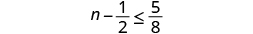Add $\frac{1}{2}$ to both sides of the inequality.Simplify.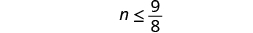Graph the solution on the number line.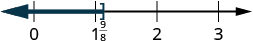Write the solution in interval notation.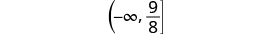Solve the inequality, graph the solution on the number line, and write the solution in interval notation.

$p-\frac{3}{4}\ge \frac{1}{6}$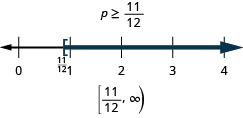Solve the inequality, graph the solution on the number line, and write the solution in interval notation.

$r-\frac{1}{3}\le \frac{7}{12}$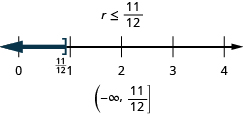## Solve inequalities using the division and multiplication properties of inequality

The Division and Multiplication Properties of Equality state that if two quantities are equal, when we divide or multiply both quantities by the same amount, the results will also be equal (provided we don’t divide by 0).

3. When Jenna spent 10 minutes on the elliptical trainer and then did circuit training for20 minutes, her fitness app says she burned 278 calories. When she spent 20 minutes onthe elliptical trainer and 30 minutes circuit training she burned 473 calories. How manycalories does she burn for each minute on the elliptical trainer? How many calories doesshe burn for each minute of circuit training?
John left his house in Irvine at 8:35 am to drive to a meeting in Los Angeles, 45 miles away. He arrived at the meeting at 9:50. At 3:30 pm, he left the meeting and drove home. He arrived home at 5:18.
p-2/3=5/6 how do I solve it with explanation pls
P=3/2
Vanarith
1/2p2-2/3p=5p/6
James
Cindy
4.5
Ruth
is y=7/5 a solution of 5y+3=10y-4
yes
James
Cindy
Lucinda has a pocketful of dimes and quarters with a value of $6.20. The number of dimes is 18 more than 3 times the number of quarters. How many dimes and how many quarters does Lucinda have? Rhonda Reply Find an equation for the line that passes through the point P ( 0 , − 4 ) and has a slope 8/9 . Gabriel Reply is that a negative 4 or positive 4? Felix y = mx + b Felix if negative -4, then -4=8/9(0) + b Felix -4=b Felix if positive 4, then 4=b Felix then plug in y=8/9x - 4 or y=8/9x+4 Felix Macario is making 12 pounds of nut mixture with macadamia nuts and almonds. macadamia nuts cost$9 per pound and almonds cost $5.25 per pound. how many pounds of macadamia nuts and how many pounds of almonds should macario use for the mixture to cost$6.50 per pound to make?
Nga and Lauren bought a chest at a flea market for $50. They re-finished it and then added a 350 % mark - up Makaila Reply$1750
Cindy
the sum of two Numbers is 19 and their difference is 15
2, 17
Jose
interesting
saw
4,2
Cindy
Felecia left her home to visit her daughter, driving 45mph. Her husband waited for the dog sitter to arrive and left home 20 minutes, or 13 hour later. He drove 55mph to catch up to Felecia. How long before he reaches her?
integer greater than 2 and less than 12
2 < x < 12
Felix
I'm guessing you are doing inequalities...
Felix
Actually, translating words into algebraic expressions / equations...
Felix
hi
Darianna
hello
Mister
Eric here
Eric
6
Cindy
He charges $125 per job. His monthly expenses are$1,600. How many jobs must he work in order to make a profit of at least \$2,400?
at least 20
Ayla
what are the steps?
Alicia
6.4 jobs
Grahame
32
Grahame
1600+2400= total amount with expenses. 4000/125= number of jobs needed to make that min profit of 2400. answer is 32
Orlando
He must work 32 jobs to make a profit
POP
32
Cindy
what is algebra
repeated addition and subtraction of the order of operations. i love algebra I'm obsessed.
Shemiah
hi
Krekar
Eric here. I'm a parent. 53 years old. I have never taken algebra. I want to learn.
Eric
I am 63 and never learned algebra
Cindy
One-fourth of the candies in a bag of M&M’s are red. If there are 23 red candies, how many candies are in the bag?
they are 92 candies in the bag
POP
92
Cindy

#### Get Jobilize Job Search Mobile App in your pocket Now!By Richley CrapoBy John GabrieliBy Prateek AshtikarBy Mary CohenBy OpenStaxBy RhodesBy Stephen VoronBy OpenStaxBy Maureen MillerBy OpenStax